# Resources tagged with: Multiplication & division

Filter by: Content type:
Age range:
Challenge level:

### There are 153 results

Broad Topics > Calculations and Numerical Methods > Multiplication & division### Repeaters

##### Age 11 to 14Challenge Level

Choose any 3 digits and make a 6 digit number by repeating the 3 digits in the same order (e.g. 594594). Explain why whatever digits you choose the number will always be divisible by 7, 11 and 13.### Why 8?

##### Age 11 to 14Challenge Level

Choose any four consecutive even numbers. Multiply the two middle numbers together. Multiply the first and last numbers. Now subtract your second answer from the first. Try it with your own. . . .### Square Subtraction

##### Age 7 to 11Challenge Level

Look at what happens when you take a number, square it and subtract your answer. What kind of number do you get? Can you prove it?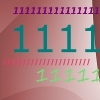### Ones Only

##### Age 11 to 14Challenge Level

Find the smallest whole number which, when mutiplied by 7, gives a product consisting entirely of ones.### Largest Product

##### Age 11 to 14Challenge Level

Which set of numbers that add to 10 have the largest product?### One O Five

##### Age 11 to 14Challenge Level

You can work out the number someone else is thinking of as follows. Ask a friend to think of any natural number less than 100. Then ask them to tell you the remainders when this number is divided by. . . .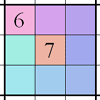### Integrated Product Sudoku

##### Age 11 to 16Challenge Level

This Sudoku puzzle can be solved with the help of small clue-numbers on the border lines between pairs of neighbouring squares of the grid.### Surprising Split

##### Age 7 to 11Challenge Level

Does this 'trick' for calculating multiples of 11 always work? Why or why not?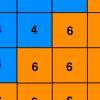### Six Ten Total

##### Age 7 to 11Challenge Level

This challenge combines addition, multiplication, perseverance and even proof.### The Genie in the Jar

##### Age 11 to 14Challenge Level

This jar used to hold perfumed oil. It contained enough oil to fill granid silver bottles. Each bottle held enough to fill ozvik golden goblets and each goblet held enough to fill vaswik crystal. . . .### Gabriel's Problem

##### Age 11 to 14Challenge Level

Gabriel multiplied together some numbers and then erased them. Can you figure out where each number was?### Multiply the Addition Square

##### Age 11 to 14Challenge Level

If you take a three by three square on a 1-10 addition square and multiply the diagonally opposite numbers together, what is the difference between these products. Why?### Product Sudoku

##### Age 11 to 16Challenge Level

The clues for this Sudoku are the product of the numbers in adjacent squares.### Missing Multipliers

##### Age 7 to 14Challenge Level

What is the smallest number of answers you need to reveal in order to work out the missing headers?### The Remainders Game

##### Age 7 to 14Challenge Level

Play this game and see if you can figure out the computer's chosen number.### Factoring Factorials

##### Age 11 to 14Challenge Level

Find the highest power of 11 that will divide into 1000! exactly.### Eminit

##### Age 11 to 14Challenge Level

The number 8888...88M9999...99 is divisible by 7 and it starts with the digit 8 repeated 50 times and ends with the digit 9 repeated 50 times. What is the value of the digit M?### Powerful Factorial

##### Age 11 to 14Challenge Level

6! = 6 x 5 x 4 x 3 x 2 x 1. The highest power of 2 that divides exactly into 6! is 4 since (6!) / (2^4 ) = 45. What is the highest power of two that divides exactly into 100!?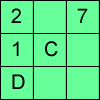### Alphabetti Sudoku

##### Age 11 to 16Challenge Level

This Sudoku requires you to do some working backwards before working forwards.### Double Digit

##### Age 11 to 14Challenge Level

Choose two digits and arrange them to make two double-digit numbers. Now add your double-digit numbers. Now add your single digit numbers. Divide your double-digit answer by your single-digit answer. . . .### Times Right

##### Age 11 to 16Challenge Level

Using the digits 1, 2, 3, 4, 5, 6, 7 and 8, mulitply a two two digit numbers are multiplied to give a four digit number, so that the expression is correct. How many different solutions can you find?### Journeys on the Gattegno Tens Chart

##### Age 5 to 11

Alf describes how the Gattegno chart helped a class of 7-9 year olds gain an awareness of place value and of the inverse relationship between multiplication and division.### Thirty Six Exactly

##### Age 11 to 14Challenge Level

The number 12 = 2^2 × 3 has 6 factors. What is the smallest natural number with exactly 36 factors?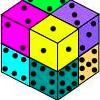### Six Numbered Cubes

##### Age 7 to 11Challenge Level

This task combines spatial awareness with addition and multiplication.### A First Product Sudoku

##### Age 7 to 14Challenge Level

Given the products of adjacent cells, can you complete this Sudoku?### Going Round in Circles

##### Age 11 to 14Challenge Level

Mathematicians are always looking for efficient methods for solving problems. How efficient can you be?### Adding All Nine

##### Age 11 to 14Challenge Level

Make a set of numbers that use all the digits from 1 to 9, once and once only. Add them up. The result is divisible by 9. Add each of the digits in the new number. What is their sum? Now try some. . . .### Funny Factorisation

##### Age 11 to 16Challenge Level

Using the digits 1 to 9, the number 4396 can be written as the product of two numbers. Can you find the factors?### Charitable Pennies

##### Age 7 to 14Challenge Level

Investigate the different ways that fifteen schools could have given money in a charity fundraiser.### Oh! Hidden Inside?

##### Age 11 to 14Challenge Level

Find the number which has 8 divisors, such that the product of the divisors is 331776.### Legs Eleven

##### Age 11 to 14Challenge Level

Take any four digit number. Move the first digit to the end and move the rest along. Now add your two numbers. Did you get a multiple of 11?### A One in Seven Chance

##### Age 11 to 14Challenge Level

What is the remainder when 2^{164}is divided by 7?### Remainders

##### Age 7 to 14Challenge Level

I'm thinking of a number. My number is both a multiple of 5 and a multiple of 6. What could my number be?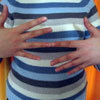### What Is the Question?

##### Age 11 to 16Challenge Level

These pictures and answers leave the viewer with the problem "What is the Question". Can you give the question and how the answer follows?### Multiples Sudoku

##### Age 11 to 14Challenge Level

Each clue in this Sudoku is the product of the two numbers in adjacent cells.### Please Explain

##### Age 11 to 14Challenge Level

Visitors to Earth from the distant planet of Zub-Zorna were amazed when they found out that when the digits in this multiplication were reversed, the answer was the same! Find a way to explain. . . .### Largest Number

##### Age 11 to 14Challenge Level

What is the largest number you can make using the three digits 2, 3 and 4 in any way you like, using any operations you like? You can only use each digit once.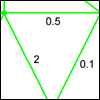### Route Product

##### Age 7 to 11Challenge Level

Find the product of the numbers on the routes from A to B. Which route has the smallest product? Which the largest?### Zargon Glasses

##### Age 7 to 11Challenge Level

Zumf makes spectacles for the residents of the planet Zargon, who have either 3 eyes or 4 eyes. How many lenses will Zumf need to make all the different orders for 9 families?### Book Codes

##### Age 7 to 11Challenge Level

Look on the back of any modern book and you will find an ISBN code. Take this code and calculate this sum in the way shown. Can you see what the answers always have in common?### Being Resilient - Primary Number

##### Age 5 to 11Challenge Level

Number problems at primary level that may require resilience.### Cows and Sheep

##### Age 7 to 11Challenge Level

Use your logical reasoning to work out how many cows and how many sheep there are in each field.### Tom's Number

##### Age 7 to 11Challenge Level

Work out Tom's number from the answers he gives his friend. He will only answer 'yes' or 'no'.### Dice and Spinner Numbers

##### Age 7 to 11Challenge Level

If you had any number of ordinary dice, what are the possible ways of making their totals 6? What would the product of the dice be each time?### Multiplication Series: Illustrating Number Properties with Arrays

##### Age 5 to 11

This article for teachers describes how modelling number properties involving multiplication using an array of objects not only allows children to represent their thinking with concrete materials,. . . .### Method in Multiplying Madness?

##### Age 7 to 14Challenge Level

Watch our videos of multiplication methods that you may not have met before. Can you make sense of them?### Ducking and Dividing

##### Age 7 to 11Challenge Level

Your vessel, the Starship Diophantus, has become damaged in deep space. Can you use your knowledge of times tables and some lightning reflexes to survive?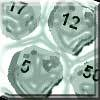### Asteroid Blast

##### Age 7 to 11Challenge Level

A game for 2 people. Use your skills of addition, subtraction, multiplication and division to blast the asteroids.### Divide it Out

##### Age 7 to 11Challenge Level

What is the lowest number which always leaves a remainder of 1 when divided by each of the numbers from 2 to 10?### Magic Potting Sheds

##### Age 11 to 14Challenge Level

Mr McGregor has a magic potting shed. Overnight, the number of plants in it doubles. He'd like to put the same number of plants in each of three gardens, planting one garden each day. Can he do it?